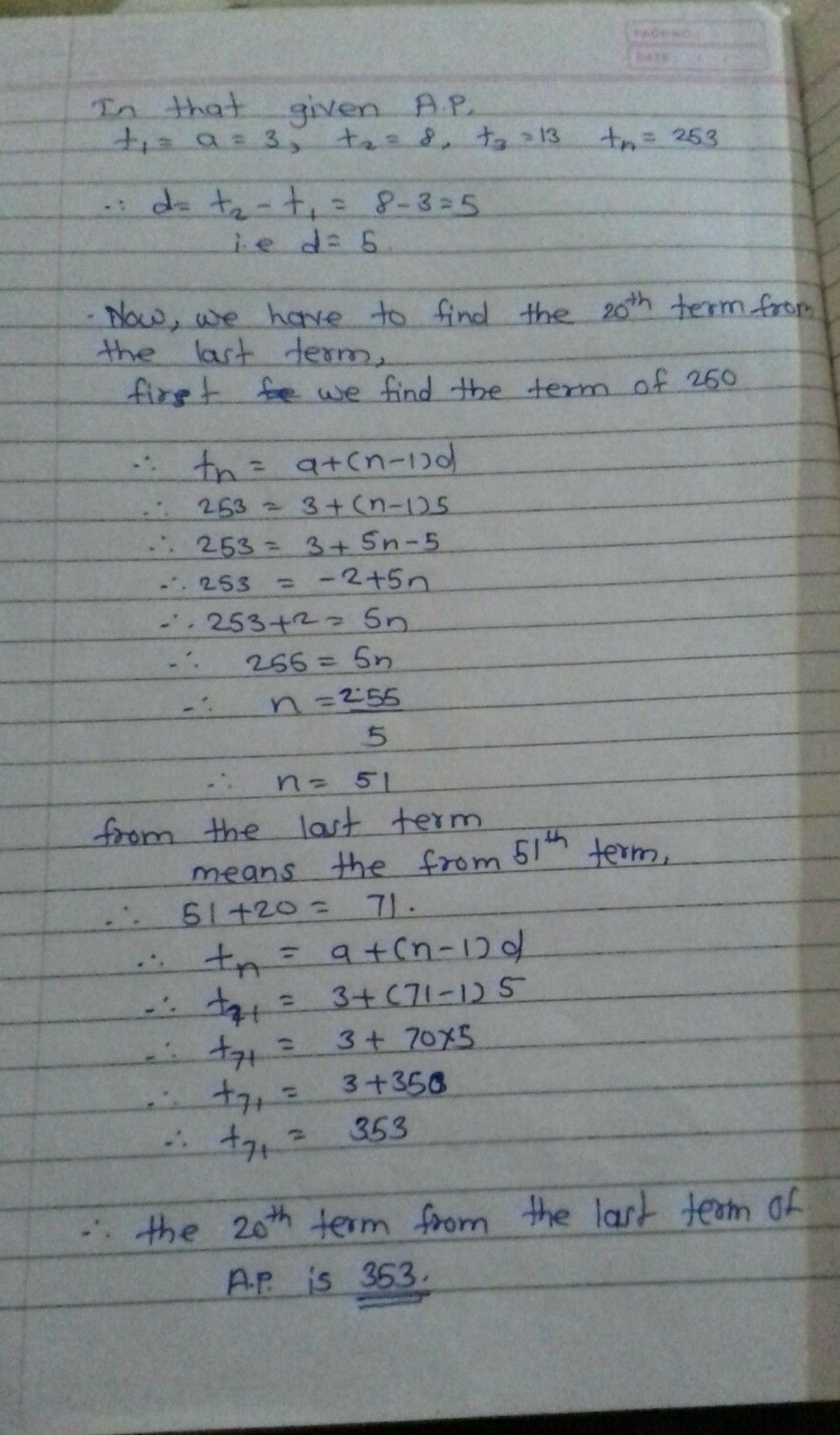Symbol
Problem$AR1m\left(M1711C$ $PR0cRPss100ys$ $1107$ 17. Find the 20th term from the last term of the $AP:3.8,13$ .. $253$ The sum of the 4th and 8th terms of $anAPis24$ and the sum of the 6th and 10th terms is 44. Find the first three terms of the $AP$ 19. Subba Rao started work in $1995$ at an annual salary $otz5000$ and received an increment of $fq200$ each year. In which year did his income reach ? $70007$ $20.$ Ramkali savedR 5 in the first week of a year and then increased her weekly savings by $q1.75$ If in the nth week, her weekly savings $bccom$ 7 $20.75$ find n. $5.4$ Sum of First n Terms of an AP Let us consider the situation again given in Section $5.1$ in which Shakila put ? $100$ into her daughter's money
Other
SolutionQanda teacher - gaurav sir